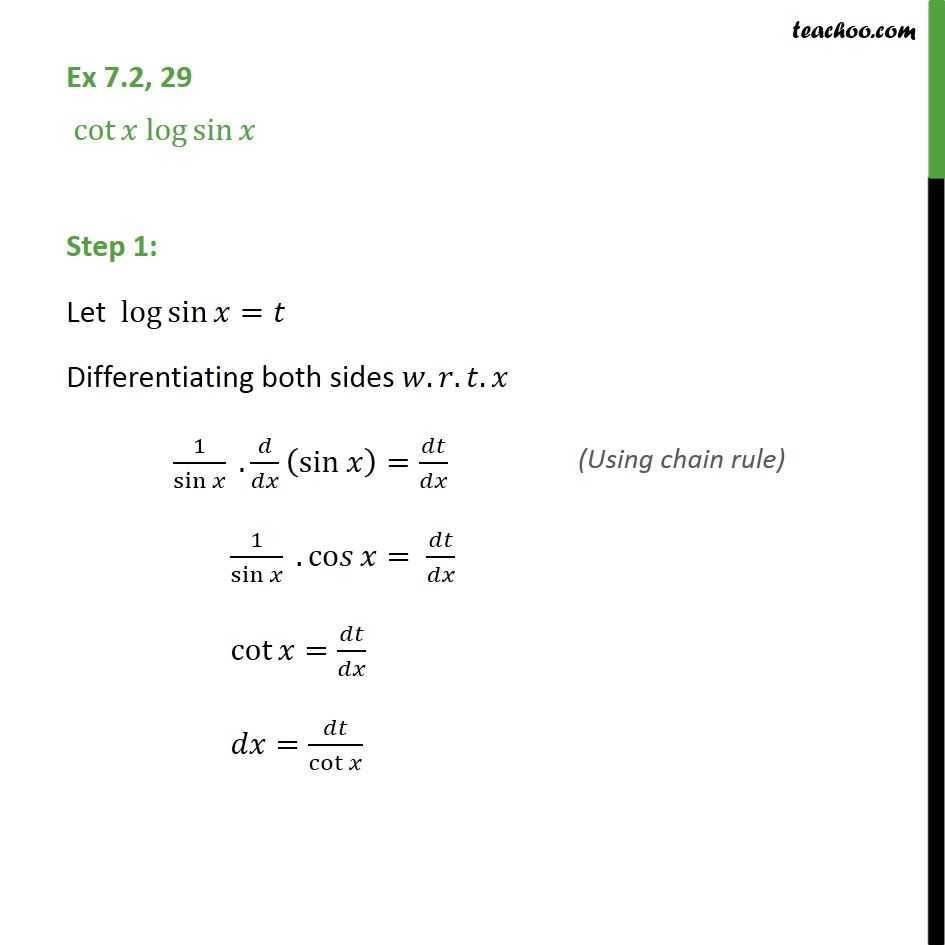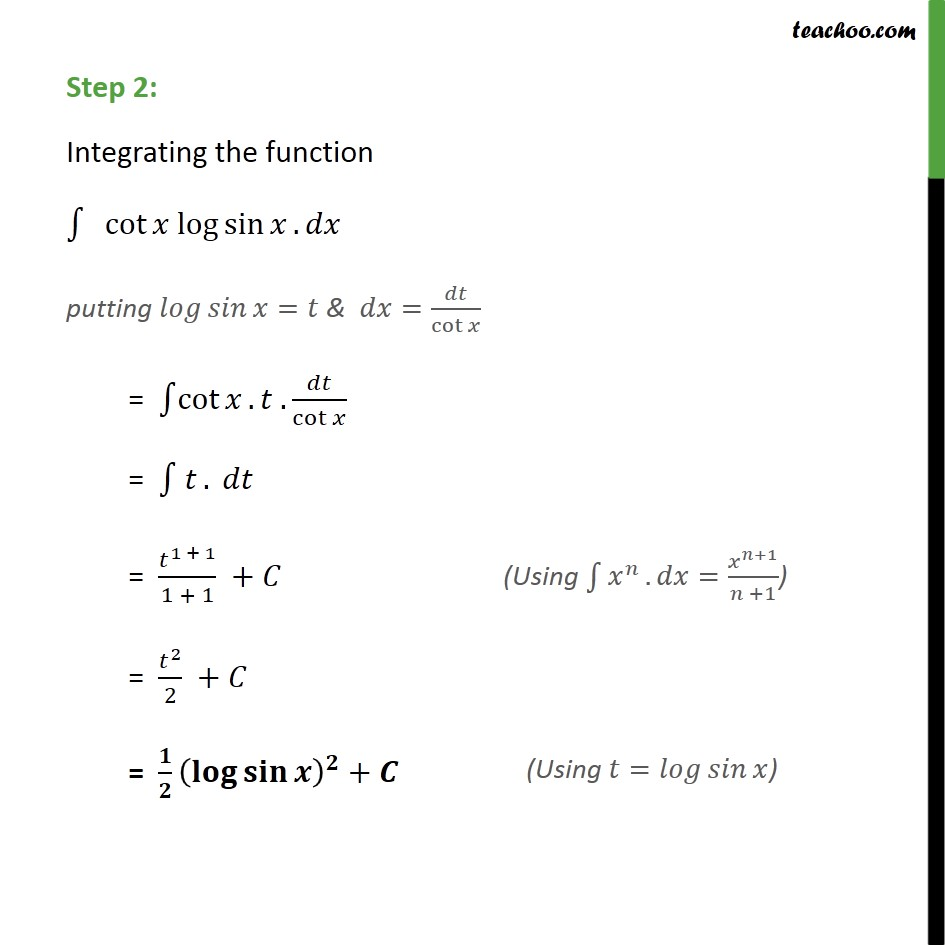1. Chapter 7 Class 12 Integrals (Term 2)
2. Concept wise
3. Integration by substitution - Trignometric - Normal

Transcript

Ex 7.2, 29 cot log sin Step 1: Let log sin = Differentiating both sides . . . 1 sin . sin = 1 sin . co = cot = = cot Step 2: Integrating the function cot log sin . putting = & = cot = cot . . cot = . = 1 + 1 1 + 1 + = 2 2 + = +